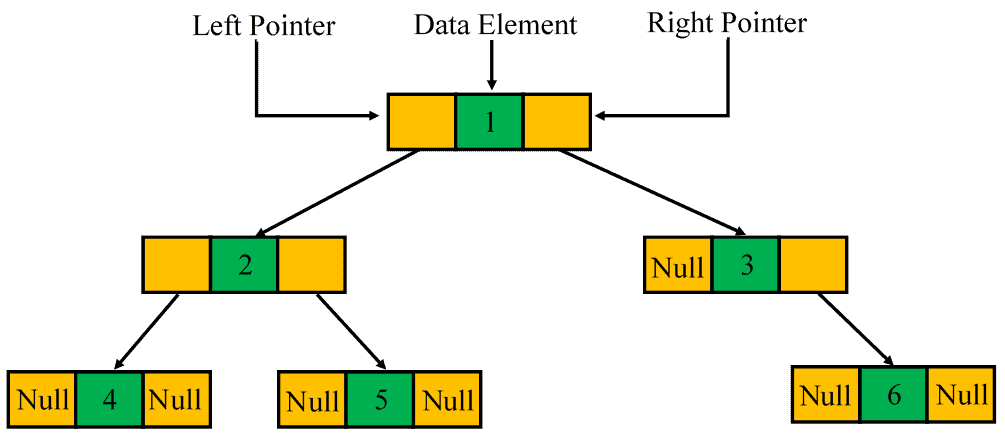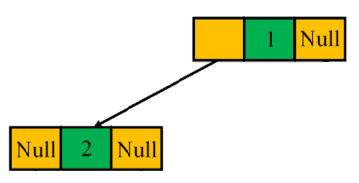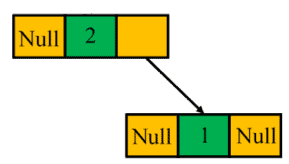## 1. Introduction

Serialization is translating a data structure or object into a sequence of bits so that it can be stored in a file or memory buffer, or transmitted across a network. In the future, we can deserialize the sequence to reconstruct the same data structure or object.

In this tutorial, we’ll introduce algorithms to serialize and deserialize a binary tree.

## 2. Binary Tree Definition

A binary tree is a hierarchal data structure in which each node has at most two children. Each node of a binary tree has 3 elements: a data element to hold the node data and two children pointers to point its left and right children. If a child node does not exist, we useto represent that:There are 3 main binary tree traversal methods: pre-order, in-order, and post-order. We can serialize and deserialize a binary tree with a combination of two methods, e.g., pre-order and in-order methods.

In this tutorial, we’ll show how to serialize and deserialize a binary tree with a single tree traversal method. For example, we can serialize a binary with pre-order traversal and deserialize it with the same method.

## 3. Serialize a Binary Tree with Pre-order Traversal

We can use the pre-order traversal algorithm to serialize a binary tree. In a pre-order binary tree traversal, we traverse the root node first. Then, we traverse its left and right subtrees, respectively. For example, we can pre-order traverse the above example tree in the order:.

When we serialize the tree, we also consider thenodes. In this case, we can useto represent anode. Therefore, the pre-order sequence becomes:.

We can use a recursive pre-order traversal algorithm to construct the serialization sequence:In this algorithm, we first serialize thenode data, then recursively serialize its left and right children. If we meet anode, we also serialize it with a special character.

If the binary tree containsnodes, the overall running time of this algorithm issince we visit each node only once. The space requirement is alsoto store the serialization sequence. Although we need extra space to store thenodes in the serialization sequence, the extra space is at mostbecause each node has at most two children.

## 4. Deserialize a Binary Tree with Pre-order Traversal

We can use the same pre-order algorithm to deserialize a sequence into a binary tree:For the simplicity of algorithm description, we use an iterator object of the serialization sequence as the input of the recursive function. Different programming languages have similar iterator support. For example, we can use an Iterator interface in Java.

The deserialization process is similar to the serialization process. We first deserialize the root node data, then recursively deserialize its left and right children. If we see a special character, we deserialize it as anode.

The overall running time and space requirement are bothsince we construct each node only once.

## 5. Serialize a Binary Tree with Post-order Traversal

Similar to the pre-order traversal, we can use use the post-order traversal algorithm to serialize a binary tree. In a post-order binary tree traversal, we first traverse its left and right subtrees respectively. Then, we visit the root node at last. We can use a recursive post-order traversal algorithm to construct the serialization sequence:In this algorithm, we first recursively serialize the left and right children. Then, we serialize the root node data in the end. The overall running time and space requirement are also.

## 6. Deserialize a Post-order Traversal Sequence

In the pre-order serialization, we put the root node at the beginning of the sequence. However, we put the root node at the end of the sequence in a post-order serialization. For example, we can post-order traverse the example tree in the order:. Therefore, the post-order traversal is a kind of reverse operation of the pre-order traversal.

To serialize a post-order traversal sequence, we can first reverse the sequence and then use a modified pre-order deserialization process to build the binary tree:In the post-order traversal, we traverse the tree in the order:

• left subtree
• right subtree
• root node

Since our deserialization process works in a backward order, we deserialize the root node first. Then, we deserialize the right subtree. Finally, we deserialize the left subtree.

The overall running time and space requirement are bothsince we construct each node only once.

## 7. In-order Traversal Binary Tree Serialization and Deserialization

We cannot use the in-order tree traversal method to serialize and deserialize a binary tree. In an in-order binary tree traversal, we traverse the left subtree first. Then, we visit the root node and traverse the right subtree. This traversal makes it hard to locate the root node in the serialized sequence.

For example, an in-order traversal of the following binary tree can produce a sequence::However, we can produce the same in-order sequence on the following binary tree:Therefore, we cannot deserialize an in-order sequence back to a binary tree as the related tree is not unique.

## 8. Conclusion

In this tutorial, we showed two algorithms, pre-order traversal and post-order traversal, to serialize and deserialize a binary tree. We also showed that the in-order traversal algorithm is not suitable for binary tree serialization and deserialization.

Finally, we confirmed that all serialization and deserialization algorithms have linear time and space complexity.